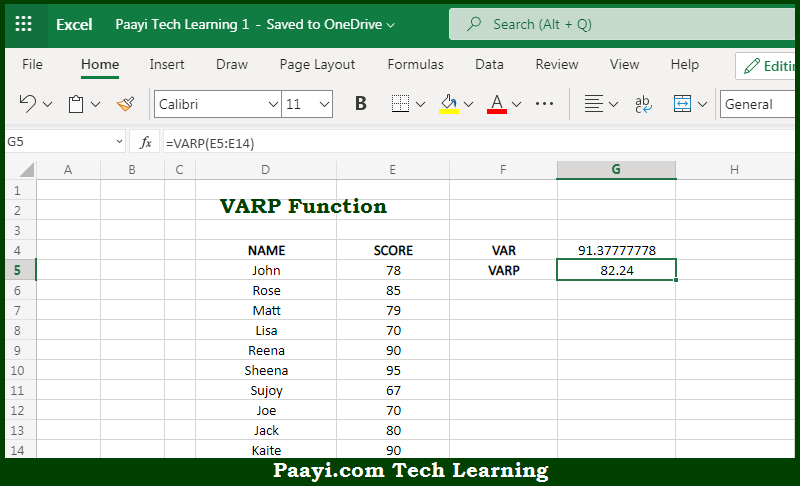# Learn How to Use Microsoft Excel VARP Function

Written by | 0 Comments | 582 Views

In this article, you will learn how to use the Microsoft Excel VARP function and its prime function in Microsoft Excel. You will also get to know the Microsoft Excel VARP function return value and syntax with the help of some examples.

Microsoft Excel VARP Function

The main purpose of the Microsoft Excel VARP function is to get the variation of the sample or population. That implies, with the help of the VARP function you can able to estimate the variance of the ENTIRE sample of a given data set or population. It should be noted that in case the data represents a sample, you can use the VAR function or the newer VAR.S function. Also, the VARP function ignores text values and logical in the references. So, with the help of the VARP function, you can able to get the variation of the entire sample or population.

Return Value of VARP Function

The return value will be the calculated variance.

Syntax of VARP Function

=VARP(number1, [number2], ...)

Where the arguments:

• number1: This is the first number or reference.
• number2: This is the second number or reference (optional).

## How to Use Microsoft Excel VARP Function?So we know that Microsoft Excel VARP function you can able to get the variation of the sample or population. That implies, with the help of the VARP function you can able to estimate the variance of the ENTIRE sample of a given data set or population. It should be noted that in case the data represents a sample, you can use the VAR function or the newer VAR.S function. Also, the VARP function ignores text values and logical in the references. So, with the help of the VARP function, you can able to get the variation of the entire sample or population.